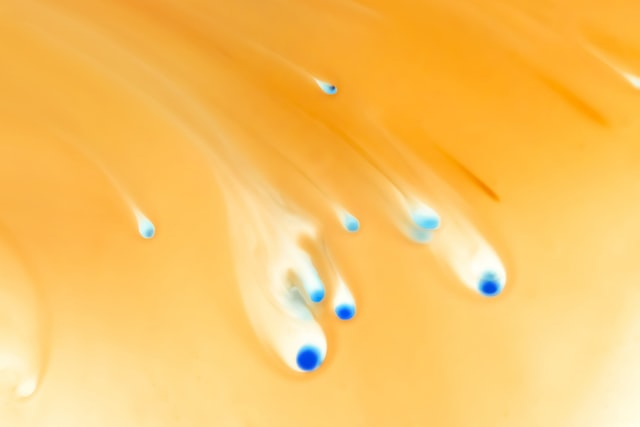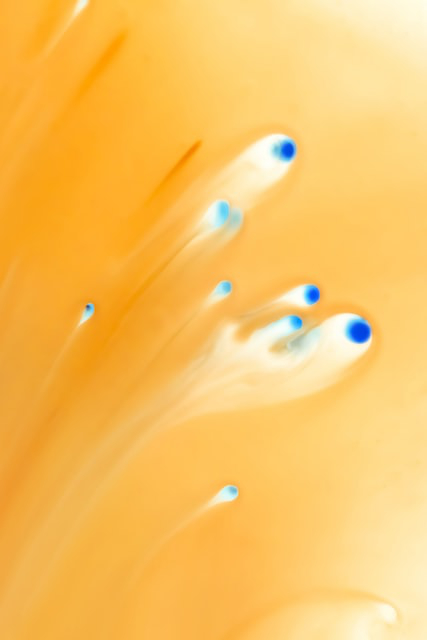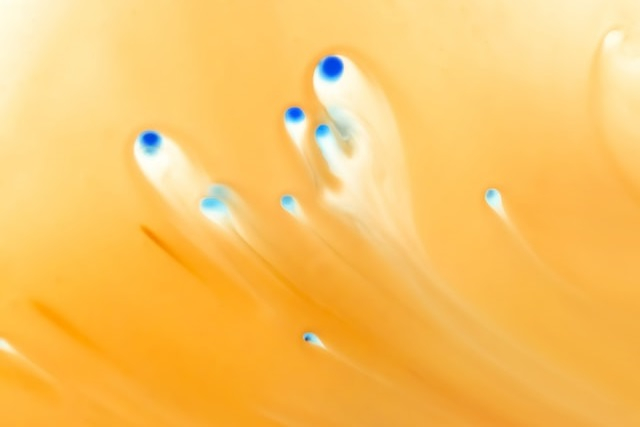# How to rotate an image with a given angle using imagerotate() function in PHP?

imagerotate() is an inbuilt function in PHP that is used to rotate an image with a given angle in degrees.

## Syntax

resource imagerotate($image,$angle, $bgd_color,$ignore_transparent = 0)

## Parameters

imagerotate() accepts four parameters, $image,$angle, $bgd_color, and$ignore_transparent.

• $image − The$image parameter returned by the imagecreatetruecolor() function. It is used to create the size of an image.

• $angle − The$angle parameter is used to hold the different rotation angles in degrees. It is used to rotate an image in the anticlockwise direction.

• $bgd_color − Holds the background color of the uncovered zone after the rotation. •$ignore_transparent − The $ignore_transparent parameter is used to set and if it is nonzero, then transparent colors are ignored. ## Return Values imagerotate() returns the image resource for the rotated image on success or it returns false on failure. ## Example 1 <?php // Assigned the image file to the variable$image_name = 'C:\xampp\htdocs\test\23.jpg';

// Load the image file using imagecreatefrompng() function
$image = imagecreatefromjpeg($image_name);

// Use imagerotate() function to rotate the image 90 degree
$img = imagerotate($image, 90, 0);

// Output the image in the browser
imagepng($img); ?> ## Input Image## Output Image## Example 2 <?php // Assigned the image file to the variable$image_name = 'C:\xampp\htdocs\test\23.jpg';

// Load the image file using imagecreatefrompng() function
$image = imagecreatefromjpeg($image_name);

// Use imagerotate() function to rotate the image 180 degree
$img = imagerotate($image, 180, 0);

// Output the image in the browser
imagepng(\$img);
?>

## OutputUpdated on: 09-Aug-2021

638 Views